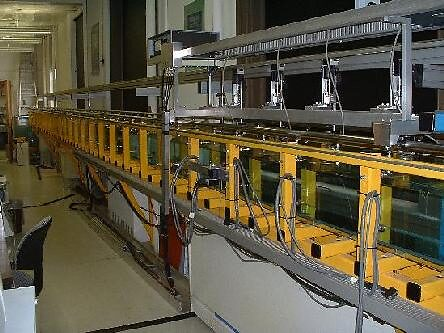# "Initial-boundary value problems arise in practice" by Dr. Hongqiu Chen### Date

Wednesday, May 20, 2015 - 13:30 to 14:30

### Location

C015, LevelC, Lab 1

### Description

Dear all,

Mathematical Soft Matter Unit (Fried Unit) would like to invite you to a Seminar by Dr. Hongqiu Chen.

-------------------------------------------
Date: Wednesday, May 20, 2015
Time: 13:30-14:30
Venue: C015, LevelC, Lab 1
-------------------------------------------

Speaker:
Dr. Hongqiu Chen
Associate Professor, Department of Mathematical Sciences,
The University of Memphis.

Title: Initial-boundary value problems arise in practice

Abstract:
The full equations for waves on the surface of an ideal fluid were
derived by Euler and Bernoulli in the 18th century. This system is not
always convenient in practice, however, model equations that formally
approximate solutions of the full problem have been developed from the
19th century up to today. It is natural to run controlled laboratory
experiments to see how well these model equations work.
Much of the mathematical theory developed for model equations is
concerned with the pure initial-value problem where the waveform is
assumed to be known everywhere at a given instant of time. In fact, it
is very difficult to determine even a fairly simple waveform at all points
in some spatial domain at one time. Much easier and far more accurate
is the determination of the wave at fixed spatial points for an interval
of time. Mathematically, this leads to initial-boundary-value problems
rather than pure initial-value problems.
Thus, in the wave tank pictured below, a flap-type wavemaker is
turned on at time t = 0 and waves are generated which propagate down
the channel. If the frequency and throw of the wavemaker are adjusted
appropriately, the waves generated are in the formal range of validity of
various model equations. The wave amplitudes are monitored at several
stations along the channel as a function of elapsed time. These measurements
are then used in the context of initial-boundary-value problems to
check the model's predictive power.
This program throws up several interesting mathematical issues. First,
one needs to understand whether or not the relevant boundary-value
problems are well posed in the classical sense of having existence and
uniqueness of solutions. The solutions should also vary only slightly
with small variations of the imposed boundary conditions - i.e. the model
should be robust. The relevant model equations are nonlinear and exact
solutions are not available even for very simple boundary data. Thus,
when such models are used in practice, a numerical scheme for approximating
its solutions must be implemented. This requires the spatial
domain to be cut off, and that in turn raises the thorny issue of whether
or not this will have an unwanted, articial eect on the solutions. Theory
relating to this issue will also be discussed.
Finally, we will discuss briefly the use of a modal decomposition to
approximate solutions of the model, a practice followed by some coastal
engineers and oceanographers.